Click to Chat

1800-1023-196

+91-120-4616500

CART 0

• 0

MY CART (5)

Use Coupon: CART20 and get 20% off on all online Study Material

ITEM
DETAILS
MRP
DISCOUNT
FINAL PRICE
Total Price: Rs.

There are no items in this cart.
Continue Shopping• Complete JEE Main/Advanced Course and Test Series
• OFFERED PRICE: Rs. 15,900
• View Details

Revision Notes on Gravitation and Projectile

Gravitation:-

Kepler’s first law (law of elliptical orbit):- A planet moves round the sun in an elliptical orbit with sun situated at one of its foci.

Kepler’s second law (law of areal velocities):- A planet moves round the sun in such a way that its areal velocity is constant.

Kepler’s third law (law of time period):- A planet moves round the sun in such a way that the square of its period is proportional to the cube of semi major axis of its elliptical orbit.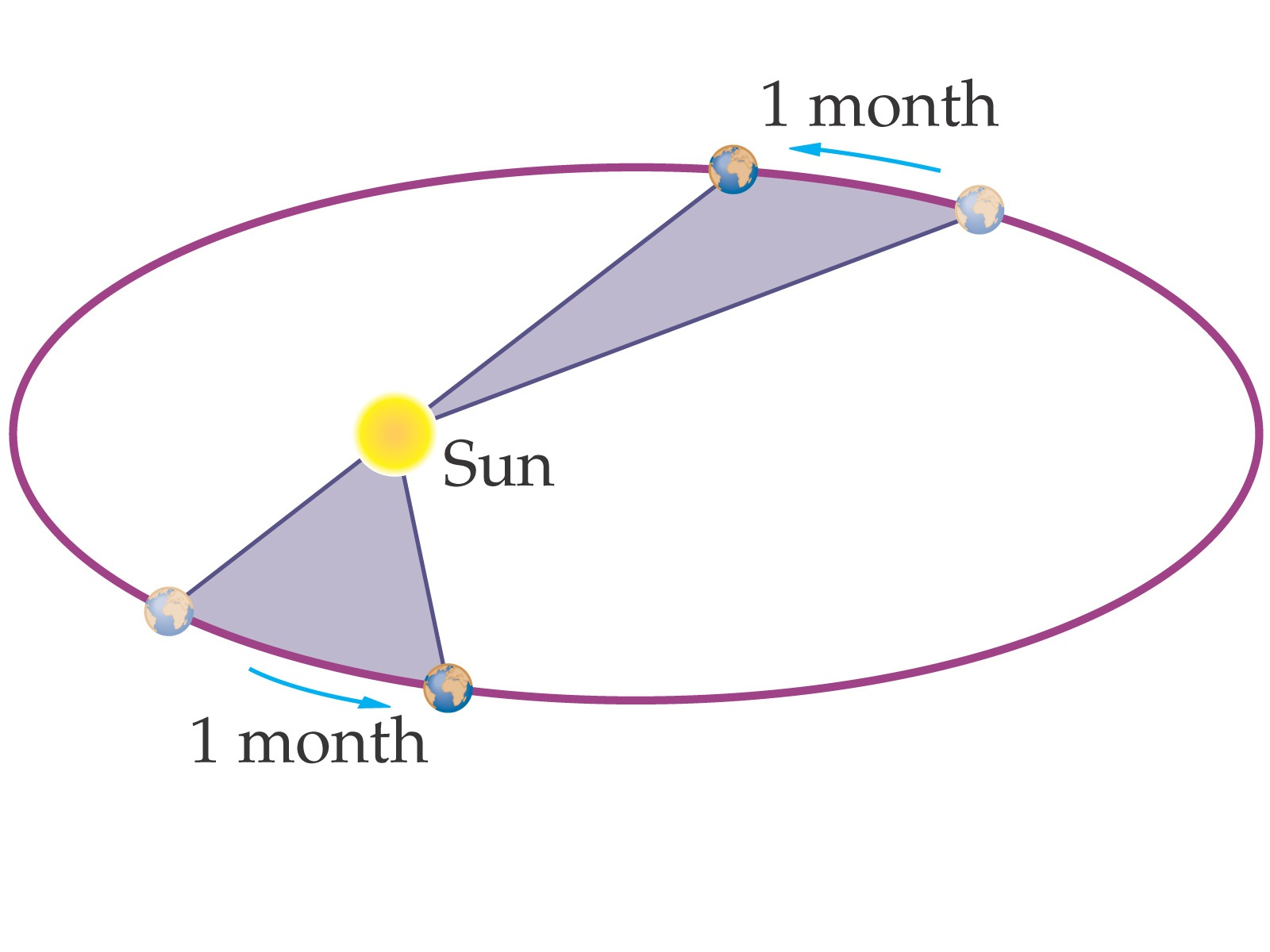T2 ∝ R3

Here R is the radius of orbit.

T2 = (4π2/GM)R 3

Newton’s law of gravitation:-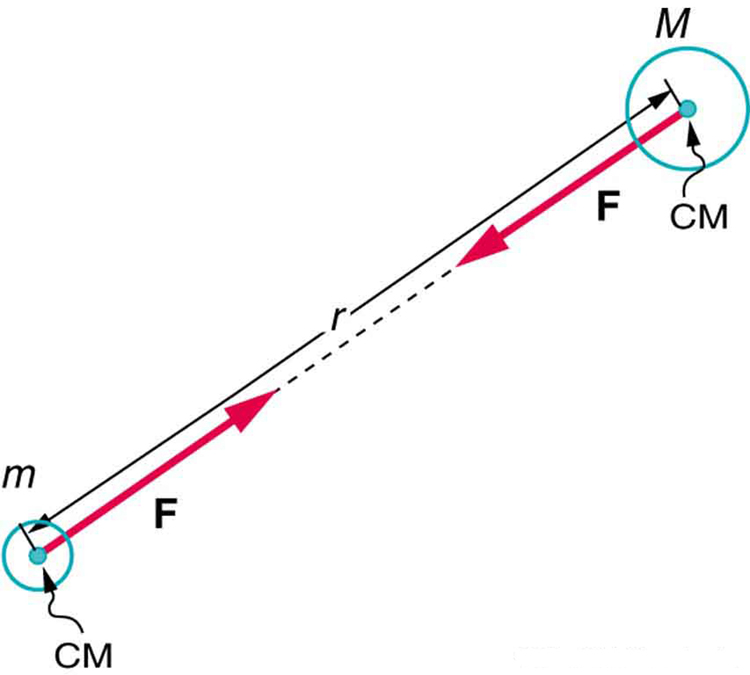Every particle of matter in this universe attracts every other particle with a forcer which varies directly as the product of masses of two particles and inversely as the square of the distance between them.

F= GMm/r2

Here, G is universal gravitational constant. G = 6.67 ´10 -11 Nm2 / kg2

Dimensional formula of G: G = Fr2/Mm =[MLT-2][L2]/[M2] = [M-1L3T-2]

Acceleration due to gravity (g):- g = GM/R2

Variation of g with altitude:- g' = g(1- 2h/R),  if h<<R. Here R is the radius of earth and h is the height of the body above the surface of earth.

Variation of g with depth:- g' = g(1- d/R). Here g' be the value of acceleration due to gravity at the depth d.

Variation with latitude:-

At poles:- θ = 90°, g' = g

At equator:- θ = 0°, g' = g (1-ω2R/g)

Here ω is the angular velocity.

As g = GMe/Re2 , therefore gpole > gequator

Gravitational Mass:- m = FR2/GM

Gravitational field intensity:-

E = F/m

= GM/r2

Weight:- W= mg

Gravitational intensity on the surface of earth (Es):-

Es = 4/3 (πRρG)

Here R is the radius of earth, ρ is the density of earth and G is the gravitational constant.

Gravitational potential energy (U):- U = -GMm/r

(a) Two particles: U = -Gm1m2/r

(b) hree particles: U = -Gm1m2/r12 – Gm1m3/r13 – Gm2m3/r23

Gravitational potential (V):- V(r) =  -GM/r

At surface of earth,

Vs=  -GM/R

Here R is the radius of earth.

Escape velocity (ve):-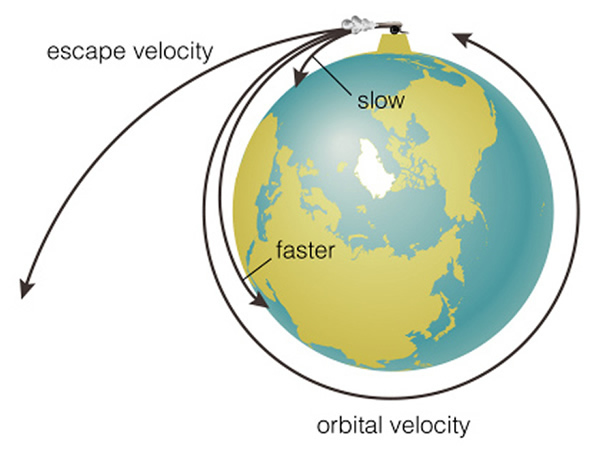It is defined as the least velocity with which a body must be projected vertically upward   in order that it may just escape the gravitational pull of earth.

ve = √2GM/R

or, ve = √2gR = √gD

Here R is the radius of earth and D is the diameter of the earth.

Escape velocity (ve) in terms of earth’s density:- ve = R√8πGρ/3

Orbital velocity (v0):-

v0 = √GM/r

If a satellite  of mass m revolves in a circular orbit around the earth of radius R and h be the height of the satellite above the surface of the earth, then,

r = R+h

So, v0 = √MG/R+h = R√g/R+h

In the case of satellite, orbiting very close to the surface of earth, then orbital velocity will be,

v0 = √gR

Relation between escape velocity ve and orbital velocity v0 :- v0= ve/√2  (if h<<R)

Time period of Satellite:- Time period of a satellite is the time taken by the satellite to complete one revolution around the earth.

T = 2π√(R+h)3/GM = (2π/R)√(R+h)3/g

If h<<R, T = 2π√R/g

Height of satellite:- h = [gR2T2/4π2]1/3 – R

Energy of satellite:-

Kinetic energy, K = ½ mv02 = ½ (GMm/r)

Potential energy, U = - GMm/r

Total energy, E = K+U

= ½ (GMm/r) + (- GMm/r)

= -½ (GMm/r)

Gravitational force in terms of potential energy:- F = – (dU/dR)

Acceleration on moon:-

gm = GMm/Rm2 = 1/6 gearth

Here Mm is the mass of moon and Rm is the radius of moon.

Gravitational field:-

(a)    Inside:-(b)   Outside:-GRAVITATIONAL POTENTIAL & FIELD DUE TO VARIOUS OBJECTS

Causing Shape

Gravitational Potential (V)

Gravitational Field (I or E)

Graph

V vs R

POINT MASS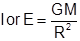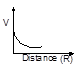AT A POINT ON THE AXIS OF RING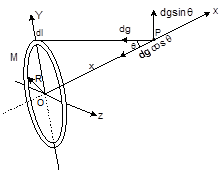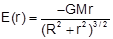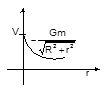ROD

1. AT AN AXIAL POINTV = –2. AT AN EQUATORIAL POINT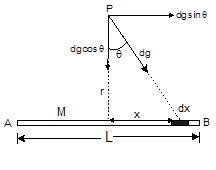CIRCULAR ARC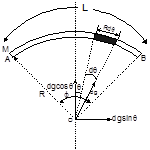HOLLOW SPHERE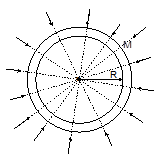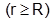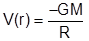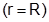SOLID SPHERE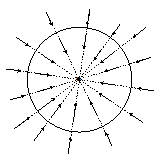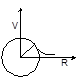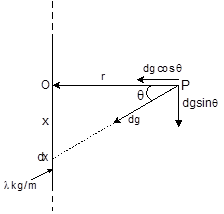V = ∞

Projectile:-

Projectile fired at angle α  with the horizontal:- If a particle having initial speed u is projected at an angle α (angle of projection) with x-axis, then,

Time of Ascent, t = (u sinα)/g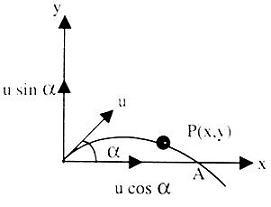Total time of Flight, T = (2u sinα)/g

Horizontal Range, R = u2sin2α/g

Maximum Height, H = u2sin2α/2g

Equation of trajectory, y = xtanα-(gx2/2u2cos2α)

Instantaneous velocity, V=√(u2+g2t2-2ugt sinα)

and

β = tan-1(usinα-gt/ucosα)

Projectile fired horizontally from a certain height:-

Equation of trajectory: x2 = (2u2/g)y

Time of descent (timer taken by the projectile to come down to the surface of earth), T = √2h/g

Horizontal Range, H = u√2h/g. Here u is the initial velocity of the body in horizontal direction.

Instantaneous velocity:-

V=√u2+g2t2

If β be the angle which V makes with the horizontal, then,

β = tan-1(-gt/u)

Projectile fired at angle α with the vertical:-

Time of Ascent, t = (u cosα)/g

Total time of Flight, T = (2u cosα)/g

Horizontal Range, R = u2sin2α/g

Maximum Height, H = u2cos2α/2g

Equation of trajectory, y = x cotα-(gx2/2u2sin2α)

Instantaneous velocity, V=√(u2+g2t2-2ugt cosα) and β = tan-1(ucosα-gt/usinα)

Projectile fired from the base of an inclined plane:-

Horizontal Range, R = 2u2 cos(α+β) sinβ/gcos2α

Time of flight, T = 2u sinβ/ gcosα

Here, α+β=θ### Course Features

• 728 Video Lectures
• Revision Notes
• Previous Year Papers
• Mind Map
• Study Planner
• NCERT Solutions
• Discussion Forum
• Test paper with Video Solution